# Methods and formulas for Goodness-of-Fit Test for Poisson

Select the method or formula of your choice.

## Estimated mean

### Formula

The mean for the Poisson distribution is estimated as:

### Computation

 Data ```2 2 3 3 2 4 4 2 1 1 1 4 4 3 0 4 3 2 3 3 4 1 3 1 4 3 2 2 1 2 0 2 3 2 3```
Category (i) Observed (Oi) Estimated mean Poisson probability (pi)
0 2 0 * 2 = 0 p0 = e-2.4 = 0.090718
1 6 1 * 6 = 6 p1 = e-2.4 * 2.4 = 0.217723
2 10 2 * 10 = 20 p2 = e-2.4 * (2.4)2/ 2! = 0.261268
3 10 3 * 10 = 30 p3 = e-2.4 * (2.4)3/ 3! = 0.209014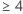7 4 * 7 = 28 p4 = 1 - (p0 + p1 +p2 + p3) = 0.221267

N = 35

Σ (i * Oi) = 84

Estimated Mean =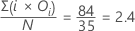### Notation

TermDescription
N sum of all observed values (O0 + O1 + ...+ Ok)
k(the number of categories) - 1
Oithe observed number of events in the ith category
piPoisson probability

## Number of categories

Minitab determines categories using the following iterative methods:

### Defining the first category

Let pi = P(X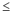xi )

Let i = 1: if N*pi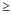2, then the first category is defined as "x 1". If N*pi < 2, then increase i by one and repeat: if N*p 22, then the first category is defined as "x 2". If N*pi < 2, increase i by one and repeat until N*pi2. Stop iterations either when this condition is first satisfied, or when xi is the third largest data value, and define the first category as "xi ". If the value of the first category is zero, then the first category is defined as "0" without the "less than or equal to" sign. The probability and expected value associated with the first category is pi and N*pi respectively. The observed value for the first category is the number of all data valuesxi .

### Defining the last category

Conceptually, defining the last category is similar to defining the first category, but Minitab works backward starting from the largest data value.

The last category is "xj ", where xj is the largest data value greater than (1 + the data value of the first category), such that the category has an expected value greater than 2. The probability and expected value for the last category are pj and N*pj respectively, and the observed value is the number of data valuesxj .

### Defining the middle categories

After determining the first and the last categories, Minitab determines the categories between them. Let "Xk" be the first category, and "Xm" is the last category. If all integers between (k, m) have expected values2, then they all constitute a middle category. If not, Minitab uses a recursive loop to group multiple adjacent integers into categories with expected values2. There are some situations, such as a data set with few observations, where the expected value of a category will be less than 2.

### Notation

TermDescription
N the total number of observations
xi the i th value in the data set after sorting it from smallest to largest
pi Poisson probability

## Poisson probability

### Formula

The Poisson probability of the i th category (i < k) is,

The Poisson probability for the last category, where i = k,

pi = 1 – (p0 + p1 + ...+ pk-1)

### Notation

TermDescription
k the number of categories
λ the estimated mean from your sample

## Expected number

### Formula

The expected number of observations in i th category is N * pi .

### Notation

TermDescription
N sample size
pi the Poisson probability associated with the i th category

## Contribution to chi-square

### Formula

Contribution of the Ith category to the chi-square value is calculated as

### Notation

TermDescription
OI the observed number of observations in the Ith category
EI the expected number of observations in the Ith category

## Test statistic

### Formula

The chi-square goodness-of-fit test statistic is calculated as,

### Notation

TermDescription
k (the number of categories) - 1
Oi the observed number of observations in the ith category
Ei the expected number of observations in the ith category

## P-value and degrees of freedom

The p-value is:

Prob (X > Test statistic)

where X follows a chi-square distribution with k - 1 degrees of freedom if you use the MEAN subcommand, or k- 2 degrees of freedom if you do not use the MEAN subcommand.

### Computation

 Data ```2 2 3 3 2 4 4 2 1 1 1 4 4 3 0 4 3 2 3 3 4 1 3 1 4 3 2 2 1 2 0 2 3 2 3```
Category (i) Observed (Oi) Estimated mean Poisson probability (pi)
0 2 0 * 2 = 0 p0 = e -2.4 = 0.090718
1 6 1 * 6 = 6 p1 = e -2.4 * 2.4 = 0.217723
2 10 2 * 10 = 20 p2 = e -2.4 * (2.4)2/ 2! = 0.261268
3 10 3 * 10 = 30 p3 = e -2.4 * (2.4)3/ 3! = 0.2090147 4 * 7 = 28 p4 = 1 - (p0 + p1 +p2 + p3 ) = 0.221267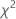= ( 0.434920 + 0.344527 + 0.080058 + 0.985114 + 0.071545) = 1.91622

k = 5= the number of categories

DF = 5- 2 = 3

p-value = P (X > 1.91622) = 0.590

### Notation

TermDescription
k the number of categories
Oi the observed number of observations in the ith category.
Ei the expected number of observations in the ith category.chi-square goodness-of-fit test statistic
DFdegrees of freedom
By using this site you agree to the use of cookies for analytics and personalized content.  Read our policy